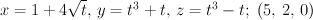# Find parametric equations for the tangent line to the curve

## Homework Statementr = <x,y,z>
r' = <x',y',z'>

## The Attempt at a Solution

I started by finding the derivatives of each part of the vector and got:

x= 2/sqrt(t) y= 3t^2+1 z= 3t^2-1

Then I plugged the point (5,2,0) into that and got (2/sqrt(5), 13, -1). This should be multiplied by t. Then by plugging in (5,2,0) back into the original line, you get (1+4sqrt(5), 10, 0). I thought that the parametric equation for this would be (1+4sqrt(5), 10, 0) + (2/sqrt(5), 13, -1)*t, but it's not working. The solution y(t) = 2+4t is already given, so I'm obviously already wrong. Any idea what I did wrong?

Mark44
Mentor

## Homework Statementr = <x,y,z>
r' = <x',y',z'>

## The Attempt at a Solution

I started by finding the derivatives of each part of the vector and got:

x= 2/sqrt(t) y= 3t^2+1 z= 3t^2-1
These would be x' = 2/sqrt(t), y'= 3t^2+1, z'= 3t^2-1

Then I plugged the point (5,2,0) into that and got (2/sqrt(5), 13, -1).
Each of these derivatives is a function of t. At the point (5, 2, 0), what's the value of t?
This should be multiplied by t. Then by plugging in (5,2,0) back into the original line, you get (1+4sqrt(5), 10, 0). I thought that the parametric equation for this would be (1+4sqrt(5), 10, 0) + (2/sqrt(5), 13, -1)*t, but it's not working. The solution y(t) = 2+4t is already given, so I'm obviously already wrong. Any idea what I did wrong?

These would be x' = 2/sqrt(t), y'= 3t^2+1, z'= 3t^2-1

Each of these derivatives is a function of t. At the point (5, 2, 0), what's the value of t?

Woops, I forgot the prime for those. What do you mean what would the value of t be? Like solve for 2/sqrt(t) = 5, 3t^2+1 = 2, and 3t^2-1 = 0 to find a t vector? Otherwise I don't see a value of t that would make the equations go through the point (5,2,0).

edit: oh nevermind t would be 1 to get to the point (5,2,0). So should I plug 1 into the derivative for each?

Mark44
Mentor
Solve for t in the equations for x, y, and z, at the point (5, 2, 0), NOT the equations for x', y', and z'. (5, 2, 0) is a point on the curve described by the parametric equations.

Ah, got it! I understand it now, I got x(t)=5+2t and z(t)=2t which were both right.